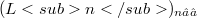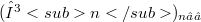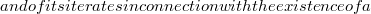﻿

### On some classes of Lototsky-Schnabl operators

#### Abstract

We study a sequence$( Ln)_{n∈ ℕ}$ of positive operators associated with a sequence$(γn)_{n∈ ℕ}$ of real numbers in the unit interval, a lower triangular stochastic matrix P and a positive projection T acting on the space of all continuous functons defined on a convex compact subset of a locally convex Hausdorff space.These operators are particular cases of the so-called Lototsky-Schnabl operators.Under suitable assumptions on(Error rendering LaTeX formula)( Ln)_{n∈ ℕ}$and of its iterates in connection with the existence of a$C0\$ - semigroup of positive contractions.

DOI Code: 10.1285/i15900932v12p1

Full Text: PDF